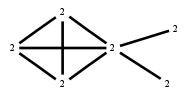# The Markov Bases Database

## G133g_bin

The binary graphical model of G133.

It is a hierarchical model of 6 variables. The dimension of the model is 19.The cardinality of the statespace is 64.

### Properties of the Markov basis

 Markov degree 2 408

 degree 2 408

The model has the following properties:

• All variables are binary.
• It is a graphical model.
• The semigroup is normal.### FILES

 Markov basis: sufficient statistics matrix: G133g_bin.mar (76.91 kb) G133g_bin.mat (3.03 kb) G133g_bin.mod (39 b) G133g_bin.tar.gz (63.76 kb)